## Frequency and Time Simulation Mode

Frequency and time simulation mode speeds up simulation of systems with a single nominal frequency by letting you increase the maximum step size for variable solvers. This mode also lets you perform phasor analysis of such systems by using the blocks in the Periodic Operators sublibrary of the Physical Signals library.

Depending on your task, you can switch between time and frequency-and-time simulation modes without modifying the model. For example, use the time simulation mode to study transient effects, and then switch to the frequency-and-time mode to perform the phasor analysis of a model.

### Speeding Up Model Simulation

Frequency-and-time equation formulation is intended for linear and linear parameter-varying (LPV) systems. It speeds up the simulation using a variable-step solver because the solver step size is no longer limited by the period of the nominal frequency.

The frequency-and-time simulation mode is based on changing the equation formulation for a physical network with a nominal frequency ω0 by dividing its variables into two categories:

• Time variables, which vary slowly relative to the nominal period 2π/ω0

• Frequency variables, which are sinusoidal and represent forced response at the nominal frequency, x = dx + axcos(ω0t) + bxsin(ω0t)

In time simulation mode, the solver step size is typically limited to a small fraction of a period of the nominal frequency. In frequency-and-time simulation mode, the representation of frequency, or fast, variables as sinusoids allows the variable solver to take much larger steps. The speeding-up effect is especially pronounced in complex machine systems that use three-phase Simscape™ Electrical™ blocks.

When you run a model in frequency-and-time simulation mode, the software automatically detects the nominal frequency and determines which of the variables are fast (frequency) and which are slow (time).

To benefit from improved performance, the time variables in the system should have slow dynamics. If time variables have time constants comparable to, or smaller than, the nominal frequency period, frequency-and-time simulation of such a system will be slow (due to the large number of timesteps required to resolve these dynamics) and possibly inaccurate. In such cases, use the time simulation mode instead.

### Variable Initialization for Frequency-and-Time Simulation

Variable initialization for frequency-and-time equation formulation follows these rules:

• For time variables and algebraic frequency variables, initialization targets and priorities are preserved.

• For dynamic frequency variables, initialization priority is switched to `None` because the solver is using the sinusoidal steady-state approximation for these variables.

### Limitations

Frequency-and-time equation formulation is intended for systems with a single nominal frequency. In other words:

• The model must have at least one sinusoidal source in its physical network.

• In case of multiple sinusoidal sources, they must all operate at the same frequency.

• Blocks outside the physical network, such as a Sine Wave block, are not considered valid sinusoidal sources.

If you try to run a frequency-and-time simulation on a model that does not meet the above criteria, you get an error message.

### Perform Sinusoidal Steady-State Analysis of a Model

This example shows how you can deploy different simulation modes on the same model, depending on the type of analysis you want to perform.

The transmission line model used in this example is built from 50 identical blocks, each block representing a single T-section segment. For more information, see Transmission Line. The model has one sinusoidal source (AC Voltage) and operates at a nominal frequency 200 MHz, which makes it a good candidate for frequency-and-time simulation.

1. Open the Transmission Line example model:

`openExample('simscape/TransmissionLineExample')`

Expand the Voltage Sensor subsystem, which consists of a Voltage Sensor block, a Solver Configuration block, and a PS-Simulink Converter block connected to the scope.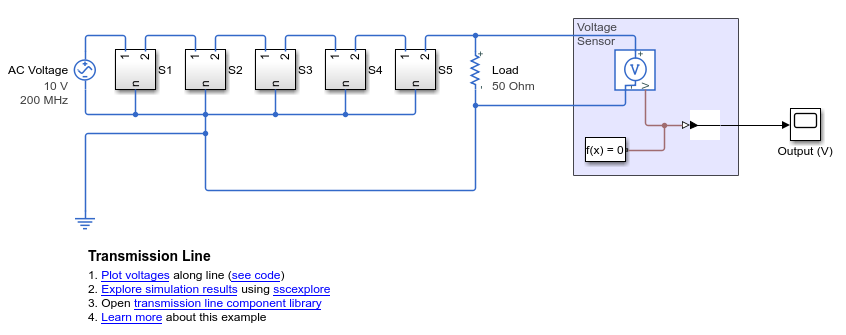2. To analyze the transient behavior of the model, run it in the time simulation mode.

Open the Solver Configuration block dialog box and verify that the Equation formulation parameter is set to `Time`. Simulate the model.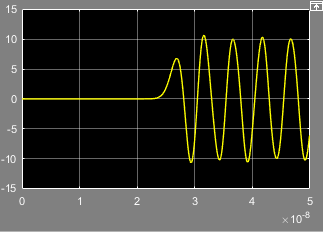You can observe the transmission delay from the simulation results.

3. To perform the phasor analysis, switch to frequency-and-time simulation mode.

Open the Solver Configuration block dialog box and set the Equation formulation parameter to ```Frequency and time```. Simulate the model.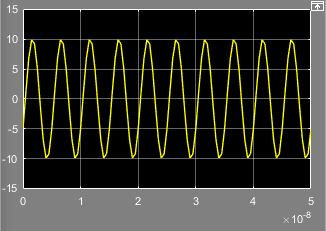Notice that in frequency-and-time mode the simulation starts in sinusoidal steady state.

4. To determine the amplitude and phase of the base frequency, connect the PS Harmonic Estimator (Amplitude, Phase) block to the voltage sensor output. Add the respective scopes.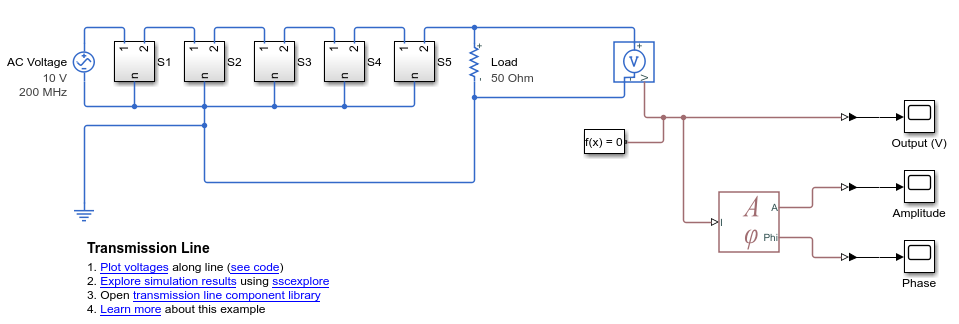5. Open the PS Harmonic Estimator (Amplitude, Phase) block dialog box and set the Base frequency parameter to `200 MHz`, to match the nominal frequency of the model. Also set the Minimum amplitude for phase detection parameter unit to `V`, to match the unit of the input signal.

6. Double-click the PS-Simulink Converter block connected to port A of the PS Harmonic Estimator (Amplitude, Phase) block. Set the Output signal unit parameter to `V`.

7. Simulate the model.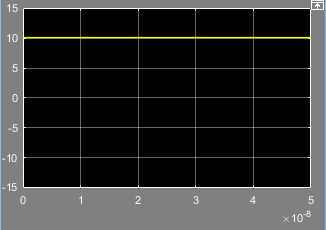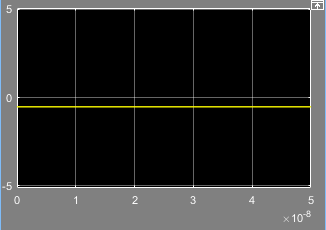8. The logged simulation data for frequency variables contains subnodes that let you examine the variable instantaneous value, amplitude, phase, and offset data separately.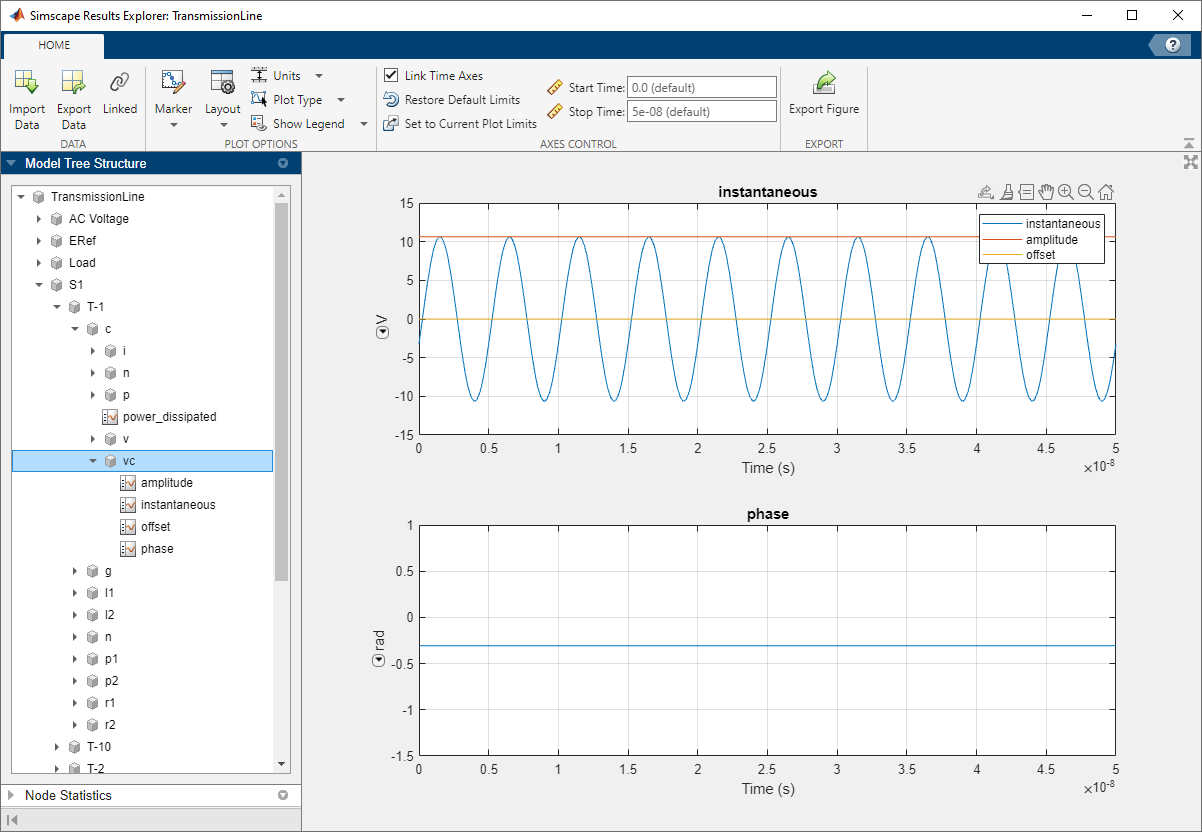Note

If you use the workflow of live-streaming the data to Simulation Data Inspector, the recorded simulation data does not contain these subnodes. To view the additional subnodes for frequency variables, clear the Record data in Simulation Data Inspector check box and rerun the simulation.